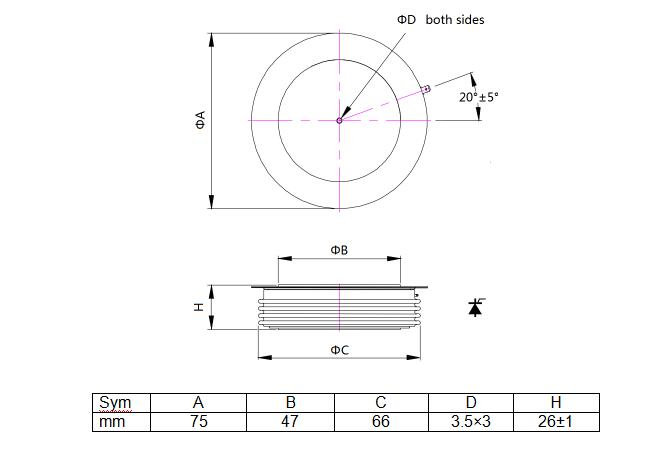# Custom capsule thyristor C712L thyristor power controller KT55CT

• YZPST-C712L

REVERSE CONDUCTING THYRISTORS

Features:

. Integrated freewheeling diode

. Optimized for low dynamic losses

Blocking - Off State

 VRRM (1) VDRM (1) VRSM (1) 2000 2000 2100

VRRM = Repetitive peak reverse voltage
VDRM = Repetitive peak off state voltage
VRSM = Non repetitive peak reverse voltage (2)

 Repetitive peak reverse leakage and off state leakage IRRM / IDRM 10 mA 70 mA (3) Critical rate of voltage rise dV/dt (4) 1000V/ µsec
"""

 Parameter Symbol Min. Max. Typ. Units Conditions Average value of on-state current IT(AV) 1185 A Sinewave,180o conduction,Tc=80oC RMS value of on-state current ITRMS 1700 A Nominal value Peak one cPSTCle surge(non repetitive) current ITSM - 18500 A A 8.3 msec (60Hz), sinusoidal wave-shape, 180o conduction, Tj = 125 oC10.0 msec (50Hz), sinusoidal wave-shape, 180o conduction, Tj = 125 oC I square t I2t 1.66x106 A2s 8.3 msec and 10.0 msec Latching current IL - mA VD = 24 V; RL= 12 ohms Holding current IH - mA VD = 24 V; I = 2.5 A Peak on-state voltage VTM 1.45 V ITM = 1000 A; Duty Cycle £ 0.01%; Tj =1 25 oC Critical rate of rise of on-statecurrent (5, 6) di/dt 800 A/ms Switching from VDRM £ 1000 V,non-repetitive Critical rate of rise of on-statecurrent (6) di/dt 200 A/ms Switching from VDRM £ 1000 V

Gating

 Parameter Symbol Min. Max. Typ. Units Conditions Peak gate power dissipation PGM 100 W tp = 40 us Average gate power dissipation PG(AV) 5 W Peak gate current IGM - A Gate current required to trigger all units IGT -120- mAmAmA VD = 6 V;RL = 3 ohms;Tj = -40 oCVD = 6 V;RL = 3 ohms;Tj = +25 oCVD = 6 V;RL = 3 ohms;Tj = +125oC Gate voltage required to trigger all units VGT -3.0- VVV VD = 6 V;RL = 3 ohms;Tj = -40 oCVD = 6 V;RL = 3 ohms;Tj = 0-125oCVD = Rated VDRM; RL = 1000 ohms;Tj = + 125 oC Peak negative voltage VGRM 20 V

Dynamic

 Parameter Symbol Min. Max. Typ. Units Conditions Delay time td - 0.7 ms ITM = 50 A; VD = Rated VDRMGate pulse: VG = 20 V; RG = 20 ohms; tr = 0.1 ms; tp = 20 ms Turn-off time (with VR = -50 V) tq 40 - ms ITM = 1000 A; di/dt = 25 A/ms;VR ³ -50 V; Re-applied dV/dt = 20 V/ms linear to 80% VDRM; VG = 0;Tj = 125 oC; Duty cPSTCle ³ 0.01% Reverse recovery charge Qrr * mC ITM = 1000 A; di/dt = 25 A/ms;VR ³ -50 V

HERMAL AND MECHANICAL CHARACTERISTICS AND RATINGS

 Parameter Symbol Min. Max. Typ. Units Conditions Operating temperature Tj -40 +125 oC Storage temperature Tstg -40 +125 oC Thermal resistance - junction to case RQ (j-c) 0.023- oC/W Double sided cooledSingle sided cooled Thermal resistamce - case to sink RQ (c-s) 0.0075- oC/W Double sided cooled *Single sided cooled * Mounting force P 22.2 26.6 kN Weight W - g About"